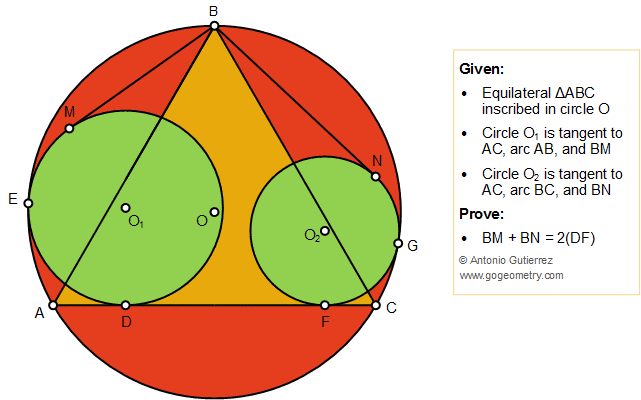## Saturday, May 18, 2019

### Geometry Problem 1434: Equilateral Triangle, Tangent Circles, Tangent Lines, Arithmetic Mean, Measurement

Geometry Problem. Post your solution in the comment box below.
Level: Mathematics Education, High School, Honors Geometry, College.

Details: Click on the figure below.#### 2 comments:

1.https://photos.app.goo.gl/hawHpVWSb5MK1TVcA

Let BO meet AC at P and circle O at Q
Since ABC is equilateral , ^(AOC)=120 => P is the midpoint of OQ
Per the result of problem 1433 we have
BN=2.PF and BM=2.PD
So BM+BN=2(PF+PD)=2.DF

2.Trough BO, make diameter BH, H on circomference,intersect with AC at K,
From 1433, BN= 2 KF,
BM=2DK,
so BM+BN= 2(DF)